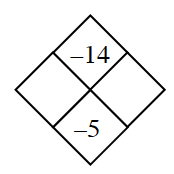### Home > CCAA8 > Chapter 2 Unit 2 > Lesson CCA: 2.3.1 > Problem2-83

2-83.

Copy and complete each of the Diamond Problems below. The pattern used in the Diamond Problems is shown at right.1.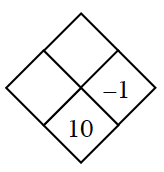$−1\: + \:? = 10$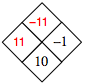1.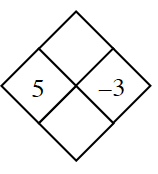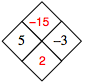1.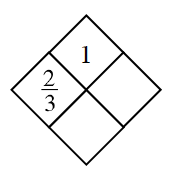$\frac{2}{3}\times y =1$

$\frac{1}{\frac{2}{3}}=x+y$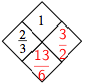1.